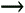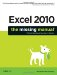Section 10.3. Math with Dates and Times

10.3. Math with Dates and Times

Because on Planet Excel, dates and times are really just special types of numbers , you can use them in calculations like addition, subtraction, and so on. In the case of dates, the most common operation is to subtract one date from another to calculate the number of days in between. For example, consider this formula:

=A2-A1

If A2 contains the value 10/30/2005, and A1 contains the value 3/20/2005, the result will be 224, which is the number of days between these two dates.

There is, however, one stumbling block when subtracting dates. As with all other types of calculations, Excel automatically applies the formatting of the source cells (those that contain the dates) to the cell that displays the result. That means that Excel will display the result of any date calculation using the Date number format.

In the previous example, Excel will display the result of 224 as August 11, 1900, which is about as helpful as saying that there are Sunday days between Wednesday and Friday. Fortunately, this problem is easy to resolve, as described in Figure 10-3.Figure 10-3. This worksheet shows one formula displayed using three different formats. The default format (shown in cell B4) displays the result as a date, which doesn't make much sense. To correct this, apply another number format. The second example (in cell B5) shows the correctly formatted result, displayed as a number. The underlying data is the same, but the display format makes much more sense. The third example (cell B6) shows the formula result displayed as an interval of time: an even 5,376 hours.

You can do other arithmetic with date values, including addition, multiplication, division, and even exponentiation. Sometimes, these things make sense; other times, they generate meaningless results. For example, the following formula calculates the interval in days between two dates, and then multiplies it by 24 to show the total number of hours (the formula assumes there are dates in cells A1 and A2):

=(A2-A1)*24

On the other hand, there are many ways you can combine date values and operators that are technically correct, but won't make much sense. One example is adding together two dates, which simply adds the two date numbers. Excel allows this sort of calculation, but you'll be hard-pressed to come up with handy uses for them.

You can perform the same calculations with dates that include time values. In this case, you simply need to remember that the fractional part of the number indicates the time. To convert a fractional day into a number of hours, just multiply it by 24, as demonstrated in the previous formula.

Note: The distinction between chronological time and time intervals (Figure 10-4) is particularly important if you want to find the difference between times that occur on two different days. For example, consider the formula =DATE(2005,1,2)-DATE(2005,1,1) . This formula calculates the interval between two dates separated by one day. To display the result as a time interval, choose the type 37:30:55 in the Format Cells dialog box (FormatCells) list of formats. Excel will correctly show the results as 24:00:00. In other words, there are 24 hours between the two dates in the formula.Figure 10-4. When performing calculations with time intervals, the Format Cells dialog box (choose FormatCells) gives you two different ways to show times. You can choose chronological time values (the top five options in the Type list), or an interval of time (the bottom option in the Type list in this figure). So which do you want? It depends on the type of calculation youre performing. For example, if you want to take an existing time, add one hour , and see what the new time will be, then display the result as a chronological time. On the other hand, if you want to subtract one time of day from another to calculate the number of hours in between, then display the results as a time interval, without the meaningless AM/PM indicator.

Of course, you can also use other Excel functions in your calculations. For example, you could take a number that represents a combined date and time value, extract just the fractional time portion, and round it to a number of whole hours using the following formula:

=ROUND((A1-INT(A1))*24, 0)

This formula works on a date in cell A1. Here's how it breaks down. The formula begins by calculating INT(A1) . This function truncates the date number, removing the fractional time portion. Then, the formula evaluates A1-INT(A1) . This leaves you with just the fractional time portion of the date. Next , multiply this fractional part by 24 (the number of hours in a day), and then rounds it to a whole number. The end result is that you extract the number of hours in a date/time value. Figure 10-5 shows some examples of date calculations similar to this one.Figure 10-5. This worksheet shows different ways to find the difference between two times.

As you'll see in the following sections, Excel has a slew of date functions that make calculations easier than this. The first calculation (=B1-B2), shown in cell B4 (In Figure 10-5), uses the default format, which incorrectly interprets the time difference as a time value. The second calculation (cell B5) uses the same formula but a better time format (the same one used in Figure 10-4). Excel displays the result as an interval of time. The next example (cell B6) uses the General format to display the result in terms of the number of fractional days. Finally, the last two examples take the additional step of multiplying the number of fractional days by 24 (to calculate fractional hours) and by 60 (to calculate fractional minutes).

Note: Remember, if you change the number format, you might end up hiding part of the date's information. For example, if you choose to display a combined date and time value using a time-only format (as shown in Figure 10-4), you won't see the date information. However, Excel will still use this date information in any calculations you perform, which might lead to unexpected data. For best results, perform all your date manipulation using the functions described later in this chapter, rather than writing your own formulas.Excel 2010: The Missing Manual
ISBN: 1449382355
EAN: 2147483647
Year: 2003
Pages: 185

Similar book on Amazon